## Excel DATEDIF function

In Excel worksheet, if you want to calculate the number of years, months, days or weeks between two given dates, the DATEDIF function in Excel may help you.

#### Syntax:

The syntax for the DATEDIF function in Excel is:

=DATEDIF(Start_Date, End_Date, Unit)

#### Arguments:

• Start_Date, End_Date: The first and last dates to calculate the difference between.
• Unit: The type of information you want to return.

Note: If the Start_date is greater than the End_date, the result will be #NUM! error value.

 Unit Returns “Y” The number of complete years between two dates. “M” The number of complete months between two dates. “D” The number of days between two dates. “MD” The difference between the days. The months and years of the dates are ignored. “YM” The difference between the days. The months and years of the dates are ignored. “YD” The difference between the days. The years of the dates are ignored.

#### Return:

Return the number of years, months, or days between two given dates.

#### Examples:

Example 1: To get the number of years between two dates:

Enter or copy the below formula into a blank cell to get the number of complete years between two given dates.

=DATEDIF(A2,B2,"y")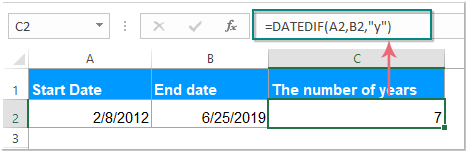Example 2: To get the number of months between two dates:

Enter or copy the following formula, and you will get the number of complete months between two given dates.

=DATEDIF(A2,B2,"m")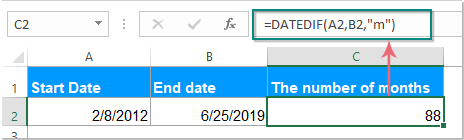Example 3: To get the number of days between two dates:

Enter or copy this formula to get the number of complete days between two given dates.

=DATEDIF(A2,B2,"d")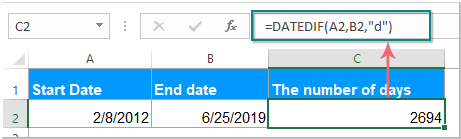Example 4: To get the number of days between two dates ignoring years and months:

Enter or copy the below formula to get the number of days ignoring years and months between two given dates.

=DATEDIF(A2,B2,"md")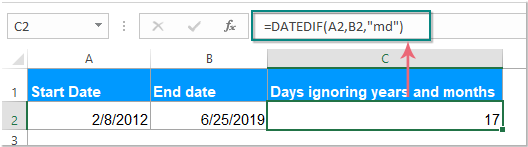Example 5: To get the number of days between two dates ignoring years only:

Enter or copy the following formula into a blank cell, and you will get the number of days excluding years only between two given dates.

=DATEDIF(A2,B2,"yd")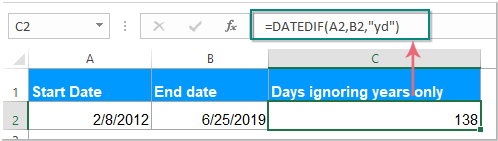Example 6: To get the number of months between two dates ignoring years and days:

Enter or copy this formula to return the number of months excluding years and days between two given dates.

=DATEDIF(A2,B2,"ym")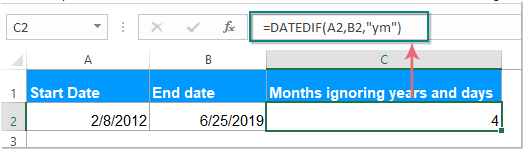Example 7: To get the number of weeks between two dates:

To return the number of weeks between two given dates, please use the following formula:

=(DATEDIF(A2,B2,"d"))/7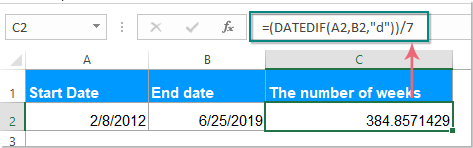Note: If you want to get the number of full weeks between the dates, please apply this formula:

=ROUNDDOWN((DATEDIF(A2, B2, "d") / 7), 0)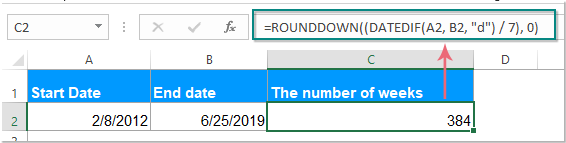Example 8: To get date difference in days, months and years between two dates:

Enter or copy the below formula to return the number of years, months and days between two given dates.

=DATEDIF(A2, B2, "y") &" years, "&DATEDIF(A2, B2, "ym") &" months, " &DATEDIF(A2, B2, "md") &" days"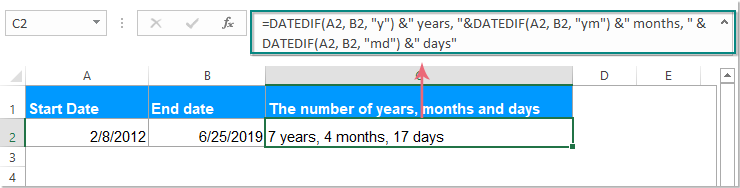Note: In the above formulas, A2 is the start date, B2 is the end date, please change them to your need.

Example 9: To calculate the ages based on birth of date:

The DATEDIF function also can help you to calculate someone's age based on the date of birth, please enter this formula:

=DATEDIF(D2,TODAY(),"y") & " Years, " & DATEDIF(D2,TODAY(),"ym") & " Months, " & DATEDIF(D2,TODAY(),"md") & " Days"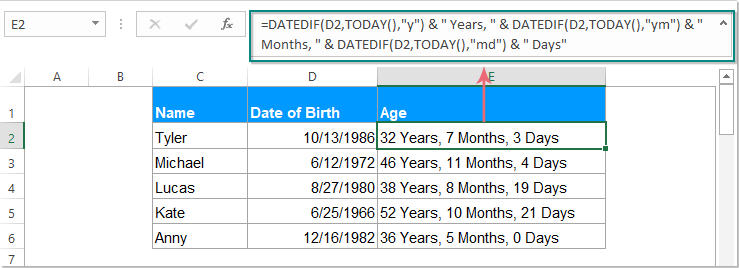Note: D2 is the cell contains the birthdate, please change the cell reference to your need.

Tips: You can also replace the cell reference in the above formulas with the date text string, such as:

=DATEDIF("1/12/2013","5/23/2018","y")

#### Relative Dateif articles:

How To Count / Calculate Weeks / Months / Years Between Two Dates In Excel?

How To Convert Birthdate To Age Quickly In Excel?

### The Best Office Productivity Tools

#### Kutools for Excel - Helps You To Stand Out From Crowd

 Popular Features: Find, Highlight or Identify Duplicates  |  Delete Blank Rows  |  Combine Columns or Cells without Losing Data  |  Round without Formula ... Super VLookup: Multiple Criteria  |  Multiple Value  |  Across Multi-Sheets  |  Fuzzy Lookup... Adv. Drop-down List: Easy Drop Down List  |  Dependent Drop Down List  |  Multi-select Drop Down List... Column Manager: Add a Specific Number of Columns  |  Move Columns  |  Toggle Visibility Status of Hidden Columns  |  Compare Columns to Select Same & Different Cells ... Featured Features: Grid Focus  |  Design View  |  Big Formula Bar  |  Workbook & Sheet Manager | Resource Library (Auto Text)  |  Date Picker  |  Combine Worksheets  |  Encrypt/Decrypt Cells  |  Send Emails by List  |  Super Filter  |  Special Filter (filter bold/italic/strikethrough...) ... Top 15 Toolsets:  12 Text Tools (Add Text, Remove Characters ...)  |  50+ Chart Types (Gantt Chart ...)  |  40+ Practical Formulas (Calculate age based on birthday ...)  |  19 Insertion Tools (Insert QR Code, Insert Picture from Path ...)  |  12 Conversion Tools (Numbers to Words, Currency Conversion ...)  |  7 Merge & Split Tools (Advanced Combine Rows, Split Excel Cells ...)  |  ... and more

Kutools for Excel Boasts Over 300 Features, Ensuring That What You Need is Just A Click Away...#### Office Tab - Enable Tabbed Reading and Editing in Microsoft Office (include Excel)

• One second to switch between dozens of open documents!
• Reduce hundreds of mouse clicks for you every day, say goodbye to mouse hand.
• Increases your productivity by 50% when viewing and editing multiple documents.
• Brings Efficient Tabs to Office (include Excel), Just Like Chrome, Edge and Firefox.